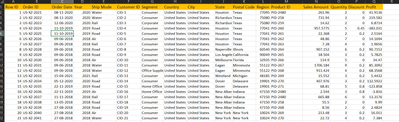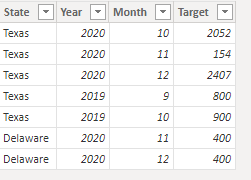cancel
Showing results for
Did you mean:Anonymous
Not applicable

## How to find the states and regions that have achieved their current year sales targets

I have a sales order table with the Order ID, Order Date, Year, Regio, State, Sales Amount, Quantity, Discount, and etc.I have another table with the sales target for a given state, in a year and month.How to find the states(Highlight) that have achieved their sales target?

Thank you. Any help would be appreciated.

1 ACCEPTED SOLUTIONCommunity Support

Hi, @Anonymous

You can try the following methods.
Sample data:

Table1:Table2:Column:

``Order Month = MONTH([Order Date])``
``````Sales Sum = CALCULATE(SUM(Table1[Sales Amount]),
ALLEXCEPT(Table1,Table1[Year],Table1[Order Month],Table1[Postal Code]))``````
``````Target = LOOKUPVALUE(Table2[Target],
Table2[Year],[Year],
Table2[Month],[Order Month],
Table2[State],[Postal Code])``````
``Result = IF([Sales Sum]>=[Target],1,0)``Is this the result you expect?

Best Regards,

Community Support Team _Charlotte

If this post helps, then please consider Accept it as the solution to help the other members find it more quickly.

2 REPLIES 2Community Support

Hi, @Anonymous

You can try the following methods.
Sample data:

Table1:Table2:Column:

``Order Month = MONTH([Order Date])``
``````Sales Sum = CALCULATE(SUM(Table1[Sales Amount]),
ALLEXCEPT(Table1,Table1[Year],Table1[Order Month],Table1[Postal Code]))``````
``````Target = LOOKUPVALUE(Table2[Target],
Table2[Year],[Year],
Table2[Month],[Order Month],
Table2[State],[Postal Code])``````
``Result = IF([Sales Sum]>=[Target],1,0)``Is this the result you expect?

Best Regards,

Community Support Team _Charlotte

If this post helps, then please consider Accept it as the solution to help the other members find it more quickly.Super User

pls share the sample data, not the screenshot. and pls also provide the expected output based on the sample data you provided.

Proud to be a Super User!